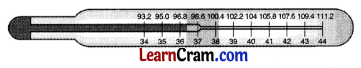# DAV Class 5 Maths Chapter 15 Worksheet 1 Solutions

The DAV Class 5 Maths Solutions and DAV Class 5 Maths Chapter 15 Worksheet 1 Solutions of Temperature offer comprehensive answers to textbook questions.

## DAV Class 5 Maths Ch 15 Worksheet 1 Solutions

Question 1.
What is the measure of hotness or coldness of a body called?
Solution:
Temperature

Question 2.
Name the instrument used for measuring temperature.
Solution:
Thermometer

Question 3.
What is the name of the thermometer used by a doctor?
Solution:
Clinical thermometerQuestion 4.
Name the two scales used for measuring temperature.
Solution:
Celsius Scale and Fahrenheit Scale.

Question 5.
What is the freezing point and the boiling point of water in the Celsius Scale?
Solution:
The freezing point of water = 0°C.
The boiling point of water = 100°C.

Question 6.
What is the freezing point and the boiling point of water in the Fahrenheit Scale?
Solution:
Freezing point = 32°F.
Boiling point = 212°F.DAV Class 5 Maths Chapter 15 Worksheet 1 Notes

• Temperature is the measure used to check hotness or coldness of a body.
• The instrument used to measure the temperature of a body is called a thermometer.• The unit used to measure the temperature is called a degree.
 Celsius Scale Fahrenheit Scale (i) Freezing point of water = 0°C (i) Freezing point of water = 32°F (ii) Boiling point of water = 100°C (ii) Boiling point of water = 212°F
• The Fahrenheit Scale is named after a German scientist G. Fahrenheit.
• The Celsius is named after a Swedish astronomer A. Celsius.
• A doctor’s thermometer is called a clinical thermometer.
• The temperature of a healthy adult is 98.6°F or 37°C.